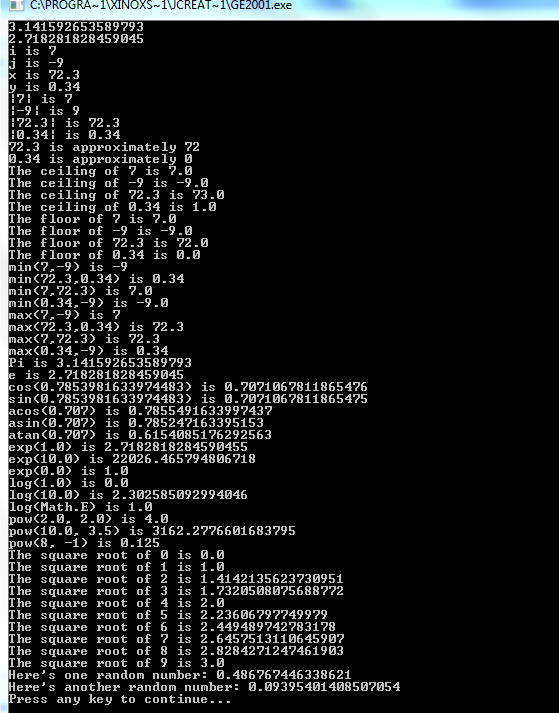# Java Programing laungage

Math Class all Function used in a program
 Previous Home Next
```//wap to uses all method of math class uses in this program

public class MathMethod
{
public static void main(String[] args)
{
int i = 7;
int j = -9;
double x = 72.3;
double y = 0.34;

System.out.println(Math.PI);

System.out.println(Math.E);

System.out.println("i is " + i);
System.out.println("j is " + j);
System.out.println("x is " + x);
System.out.println("y is " + y);

// The absolute value of a number is equal to
// the number if the number is positive or
// zero and equal to the negative of the number
// if the number is negative.

System.out.println("|" + i + "| is " + Math.abs(i));
System.out.println("|" + j + "| is " + Math.abs(j));
System.out.println("|" + x + "| is " + Math.abs(x));
System.out.println("|" + y + "| is " + Math.abs(y));

// Truncating and Rounding functions
// You can round off a floating point number
// to the nearest integer with round()

System.out.println(x + " is approximately " + Math.round(x));
System.out.println(y + " is approximately " + Math.round(y));

// The "ceiling" of a number is the
// smallest integer greater than or equal to
// the number. Every integer is its own
// ceiling.

System.out.println("The ceiling of " + i + " is " + Math.ceil(i));
System.out.println("The ceiling of " + j + " is " + Math.ceil(j));
System.out.println("The ceiling of " + x + " is " + Math.ceil(x));
System.out.println("The ceiling of " + y + " is " + Math.ceil(y));

// The "floor" of a number is the largest
// integer less than or equal to the number.
// Every integer is its own floor.

System.out.println("The floor of " + i + " is " + Math.floor(i));
System.out.println("The floor of " + j + " is " + Math.floor(j));
System.out.println("The floor of " + x + " is " + Math.floor(x));
System.out.println("The floor of " + y + " is " + Math.floor(y));

// Comparison operators
// min() returns the smaller of the two arguments you pass it

System.out.println("min(" + i + "," + j + ") is " + Math.min(i,j));
System.out.println("min(" + x + "," + y + ") is " + Math.min(x,y));
System.out.println("min(" + i + "," + x + ") is " + Math.min(i,x));
System.out.println("min(" + y + "," + j + ") is " + Math.min(y,j));

// There's a corresponding max() method
// that returns the larger of two numbers

System.out.println("max(" + i + "," + j + ") is " + Math.max(i,j));
System.out.println("max(" + x + "," + y + ") is " + Math.max(x,y));
System.out.println("max(" + i + "," + x + ") is " + Math.max(i,x));
System.out.println("max(" + y + "," + j + ") is " + Math.max(y,j));

// The Math library defines a couple
// of useful constants:

System.out.println("Pi is " + Math.PI);
System.out.println("e is " + Math.E);

// Trigonometric methods
// All arguments are given in radians
// Convert a 45 degree angle to radians

double angle = 45.0 * 2.0 * Math.PI/360.0;
System.out.println("cos(" + angle + ") is " + Math.cos(angle));
System.out.println("sin(" + angle + ") is " + Math.sin(angle));

// Inverse Trigonometric methods
// All values are returned as radians

double value = 0.707;
System.out.println("acos(" + value + ") is " + Math.acos(value));
System.out.println("asin(" + value + ") is " + Math.asin(value));
System.out.println("atan(" + value + ") is " + Math.atan(value));

// Exponential and Logarithmic Methods
// exp(a) returns e (2.71828...) raised
// to the power of a.

System.out.println("exp(1.0) is " + Math.exp(1.0));
System.out.println("exp(10.0) is " + Math.exp(10.0));
System.out.println("exp(0.0) is " + Math.exp(0.0));

// log(a) returns the natural
// logarithm (base e) of a.

System.out.println("log(1.0) is " + Math.log(1.0));
System.out.println("log(10.0) is " + Math.log(10.0));
System.out.println("log(Math.E) is " + Math.log(Math.E));

// pow(x, y) returns the x raised
// to the yth power.

System.out.println("pow(2.0, 2.0) is " + Math.pow(2.0,2.0));
System.out.println("pow(10.0, 3.5) is " + Math.pow(10.0,3.5));
System.out.println("pow(8, -1) is " + Math.pow(8,-1));

// sqrt(x) returns the square root of x.
for (i=0; i < 10; i++)
{
System.out.println("The square root of " + i + " is " + Math.sqrt(i));
}

// Finally there's one Random method
// that returns a pseudo-random number
// between 0.0 and 1.0;

System.out.println("Here's one random number: " + Math.random());
System.out.println("Here's another random number: " + Math.random());
}
}```

### output :Previous Home Next